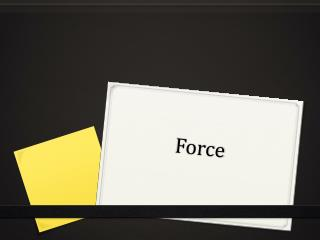DownloadDownload PresentationForce

# Force

Télécharger la présentation## Force

- - - - - - - - - - - - - - - - - - - - - - - - - - - E N D - - - - - - - - - - - - - - - - - - - - - - - - - - -
##### Presentation Transcript

1. Force

2. Unit of Force • Force is measured in Newtons (N) • 1 Newton (N) is the amount of force required to accelerate a 1 kg object at a rate of 1 m/s2

3. Newton’s Three Laws • I. Every object in a state of uniform motion tends to remain in that state of motion unless an external force is applied to it. • II. The relationship between an object's mass m, its acceleration a, and the applied force F is F = ma. • III. For every action there is an equal and opposite reaction.

4. What forces can do to an object • 1. Can cause an object to start moving or increase the speed of an already moving object. This is called Acceleration. • 2. Can cause an object to stop moving or slow down the speed of a moving object. This is called Deceleration. • 3. Can cause a moving object to change direction.

5. Types of Forces • Gravitational • Electromagnetic • Frictional • Strong and Weak Nuclear

6. Gravitational Forces • Force of attraction between all objects as a result of their masses and distances between them. • The higher the masses and the shorter the distances between two objects, the greater the force of gravity. • The smaller the masses and the larger the distances between two objects, the weaker the force of gravity.

7. Gravity exists between ANY two objects

8. Why things fall to the ground • When we drop an object, the force of gravity is strong because the earth has a large mass and is close by. The object gets pulled to the ground. • Near the Earth’s surface, objects accelerate to the ground at a rate of 9.8 m/s2, regardless of their masses.

9. The gravitational force of the Earth decreases as you move away from the planet. • Different planets and stars will have different forces of gravity at their surfaces due to their different masses. The bigger the object the greater the force of gravity. • Gravitational forces also explain the Earth’s tides

10. Mass vs. Weight • Mass is a measure of the quantity of matter in an object. This does not depend on where the object is. • Weight is a measure of the gravitational force acting on an object. This DOES depend on where the object is.

11. Acceleration • Acceleration = Change of velocity Time Taken • Example – A F1 car starts at 0 m/s and gets to 27 m/s in 3 seconds, what is the F1’s acceleration?A = 27m/s – 0m/s = 9m/s2 3 s

12. A useful formula Weight = Gravitational force = Mass X gravitational field intensity OR w = Fg = mg or F=ma m is mass measured in kilograms (kg) g gravitational field intensity measured in Newtons/kilogram (N/kg); this is also known as acceleration. F is the force measured in Newtons (N)

13. Example • What is the gravitational force (weight) of a 60 kg astronaut on the earth’s surface? Intensity of gravitation on earth is 9.8 N/kg F = mg F = 60 X 9.8 F = 588 N

14. Example • What is the gravitational force (weight) of a 60 kg astronaut on the Moon’s surface? F=mg F= 60 X 1.67 (force of gravity on the Moon) F= 100.2 N